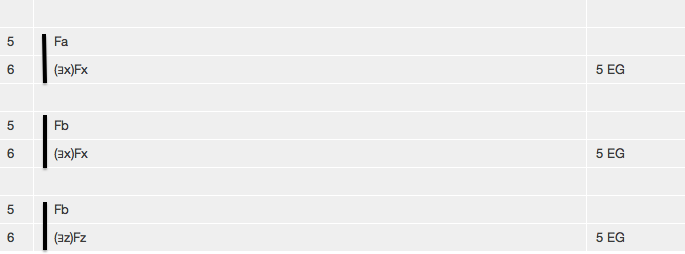# Tutorial 20: Simplified Existential Generalization

Logical System

2013

### The Tutorial

There is a rule for adding a Existential Quantifier. This permits the step illustrated by the following proof fragments.To understand what this rule permits, it helps to think about it as though you were going backwards from line 6 to line 5. Take the scope of line 6, which is Fx, Fx, and Fz respectively, then substitute a term for free occurrences of the variable of quantification-- you should be able to get line 5, and indeed you can.

Sad to say, like Universal Instantiation, there is a rather sophisticated restriction on this rule-- we will come to this in Tutorial 23.

#### The Simplified Rule of Existential Generalization EG

If a derivation contains a line of the form

n. <formula>[<term>/<variable>]> <any justification>

then a line of the form

(∃<variable>)<formula> 'n EG'

may be added to the derivation, provided that <variable> is new to <formula>.

## Exercise to accompany Predicate Tutorial 10

### Exercise 1 (of 1)

Derive the following

a) Fb∴(∃x)Fx
b) Fa∴(∃y)Fy
c) Fa∴(∃x)Fa
d) Fa,Ga∴(∃x)(Fx∧Gx)
e) Fa,Ga∴(∃x)(Fx∧Ga)
f) Fa,Ga∴(∃x)(Fa∧Gx)
g) Fa,Ga∴(∃x)(Fx)∧Ga

If you decide to use the web application for the exercises you can launch it from here Deriver [Gentzen] — username 'logic' password 'logic'. Then either copy and paste the above formulas into the Journal or use the Deriver File Menu to Open Web Page with this address https://softoption.us/test/easyDeriver/CombinedExercisesEasyDGentzen.html .

Preferences

You may need to set some Preferences for this.

• you can check that the parser is set to gentzen.